## Formula (5)

Friday, 04 May 2018 06:32

### Remove Formulas from Cells but Keep Values in Excel in C#

Written by support iceblue

Formulas are commonly used in Excel to calculate data, at some point, we may want to remove these formulas from Excel but keep their calculated values. This article is going to demonstrate how to remove formulas from cells but keep the values using Spire.XLS.

The sample file we used for demonstration: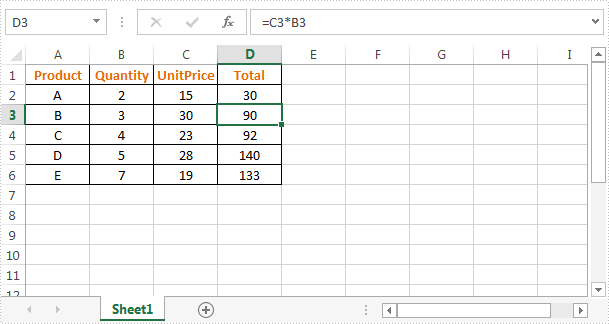Detail steps:

Step 1: Instantiate a Workbook object and load the excel file.

```Workbook workbook = new Workbook();
```

Step 2: Remove formulas from cells and keep the calculated values.

```//Loop through worksheets
foreach (Worksheet sheet in workbook.Worksheets)
{
//Loop through cells
foreach (CellRange cell in sheet.Range)
{
//If the cell contain formula, get the formula value, clear cell content, and then fill the formula value into the cell
if (cell.HasFormula)
{
Object value = cell.FormulaValue;
cell.Clear(ExcelClearOptions.ClearContent);
cell.Value2 = value;
}
}
}
```

Step 3: Save the file.

```workbook.SaveToFile("DeleteFormula.xlsx", ExcelVersion.Version2013);
```

Screenshot: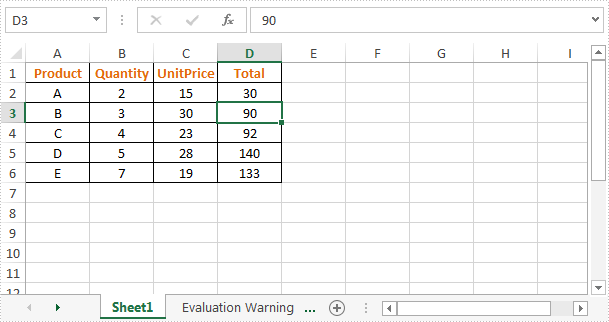Full code:

```//Instantiate a Workbook object
Workbook workbook = new Workbook();

//Loop through worksheets
foreach (Worksheet sheet in workbook.Worksheets)
{
//Loop through cells
foreach (CellRange cell in sheet.Range)
{
//If the cell contain formula, get the formula value, clear cell content, and then fill the formula value into the cell
if (cell.HasFormula)
{
Object value = cell.FormulaValue;
cell.Clear(ExcelClearOptions.ClearContent);
cell.Value2 = value;
}
}
}

//Save the file
workbook.SaveToFile("DeleteFormula.xlsx", ExcelVersion.Version2013);
```
Monday, 09 December 2013 02:29

### Create and Apply Excel Named Range in C#

Written by ring zhong

Named range is always used in formulas when processing data in Excel. Spire.XLS can meet your need here in dealing with named ranges. Spire.XLS can create a named range, edit a named range and use named ranges in formulas.

In this article, we will introduce you how to create and apply named ranges through C# code.

Call the method Add to create a new named range. The parameter NewNamedRange is the name of the named range. Then use the property RefersToRange to set the range of the named range.

[C#]
```INamedRange NamedRange = workbook.NameRanges.Add("NewNamedRange");
NamedRange.RefersToRange = sheet.Range["D2:D19"];
```

Add a formula that uses named range NewNamedRange.

[C#]
```sheet.Range["D20"].Formula = "=SUM(NewNamedRange)";
```

Edit the property RefersToRange to change the range of named range NewNamedRange.

[C#]
```INamedRange NamedRange1 = workbook.NameRanges.GetByName("NewNamedRange");
IXLSRange range = NamedRange1.RefersToRange;
range = range.Worksheet.Range["D2:D8"];
NamedRange1.RefersToRange = range;
```

PS: Since the formula uses NewNamedRange, the change of NewNamedRange affects the value of sheet.Range[“20”].

Here is the full code and effect screenshot:

[C#]
```static void Main(string[] args)
{
Workbook workbook = new Workbook();
Worksheet sheet = workbook.Worksheets;

NamedRange.RefersToRange = sheet.Range["D2:D19"];

sheet.Range["D20"].Formula = "=SUM(NewNamedRange)";

INamedRange NamedRange1 = workbook.NameRanges.GetByName("NewNamedRange");
IXLSRange range = NamedRange1.RefersToRange;
range = range.Worksheet.Range["D2:D8"];
NamedRange1.RefersToRange = range;

workbook.SaveToFile(@"..\..\result.xlsx", ExcelVersion.Version2007);
System.Diagnostics.Process.Start(@"..\..\result.xlsx");
}
```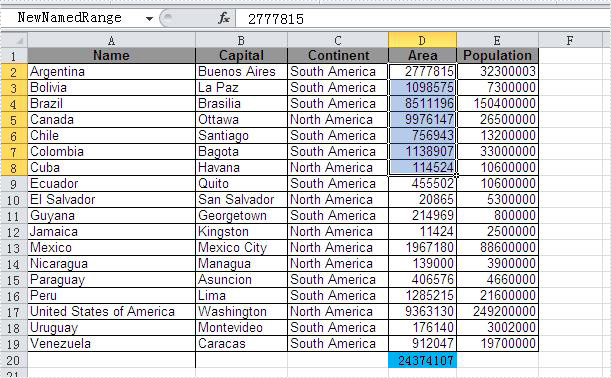Tuesday, 03 September 2013 02:08

### Background

Excel is widely used to organize data manipulations like arithmetic operations. Excel provides many built-in functions which automate a number of types of calculation. Functions are pre-programmed formulate for example, the square-root function, trigonometric functions, logarithms etc. Excel has more than 300 functions covering a range of statistical, mathematical, financial and logical operations. There is no doubt that using a function offers a shortcut method.

### Calculate Formulas in XLS Document

Microsoft Excel is a powerful tool which has many uses, the most basic feature of which is performing functions. The aim of this article is to help you perform simple arithmetic operations on values in programming by using excel functions. Spire.Xls for .NET can help you easily create a new excel document or load an existing excel document into program, and calculate data of designated cell by function. Applied in Console platform, WinForm and Asp.net, It provide different types of mathematical functions, statistical functions , logic functions ,and string functions to calculate data with C# codes.

The following is the method example of using Console application to show how Spire.XLS for .NET realizes the calculation formula:

Step 1: Build a console application, and add spire.XLS.dll, Spire.Common.dll assembly.

Step 2: Instantiate an object of Spire.Xls.WorkBook, and add a “WorkSheet” in WorkBook object.

[C#]
```Workbook workbook = new Workbook();
Worksheet sheet = workbook. Worksheets;
```

Step 3: Set the value and format in Cell A1 and Cell A3.veiwing the C# Code.

[C#]
```//set Column A, B, C width
sheet.SetColumnWidth(1, 32);
sheet.SetColumnWidth(2, 16);
sheet.SetColumnWidth(3, 16);

// Set value of Cell A1
sheet.Range[currentRow++, 1].Value = "Examples of formulas :";
// Set value of Cell A2.
sheet.Range[++currentRow, 1].Value = "Test data:";

// Set text format Of Cell A1
CellRange range = sheet.Range["A1"];
range.Style.Font.IsBold = true;
range.Style.FillPattern = ExcelPatternType.Solid;
range.Style.KnownColor = ExcelColors.LightGreen1;
range.Style.Borders[BordersLineType.EdgeBottom].LineStyle = LineStyleType.Medium;
```

Step 4: Set some cells value and then to sum up some cells data and the results will be displayed in one of the cells.

[C#]
```sheet.Range[currentRow, 2].NumberValue = 7.3;
sheet.Range[currentRow, 3].NumberValue = 5;
sheet.Range[currentRow, 4].NumberValue = 8.2;
sheet.Range[currentRow, 5].NumberValue = 4;
sheet.Range[currentRow, 6].NumberValue = 3;
sheet.Range[currentRow, 7].NumberValue = 11.3;
//Create arithmetic expression string about cells

currentFormula = "=Sheet1!\$B\$3 + Sheet1!\$C\$3+Sheet1!\$D\$3+Sheet1!\$E\$3+Sheet1!\$F\$3+Sheet1!\$G\$3";
formulaResult = workbook.CaculateFormulaValue(currentFormula);
value = formulaResult.ToString();
sheet.Range[currentRow, 2].Value = value;
```

Step 5: Respectively set value and text format of Cell A4, B4.

[C#]
```sheet.Range[++currentRow, 1].Value = "Formulas"; ;
sheet.Range[currentRow, 2].Value = "Results";
range = sheet.Range[currentRow, 1, currentRow, 2];
range.Style.Font.IsBold = true;
range.Style.KnownColor = ExcelColors.LightGreen1;
range.Style.FillPattern = ExcelPatternType.Solid;
range.Style.Borders[BordersLineType.EdgeBottom].LineStyle = LineStyleType.Medium;
```

Step 6: Realize calculation simple expression.

[C#]
```// Create arithmetic tables enclosed type string
currentFormula = "=33*3/4-2+10";
sheet.Range[++currentRow, 1].Text = currentFormula;
// Caculate arithmetic expression
formulaResult = workbook.CaculateFormulaValue(currentFormula);
value = formulaResult.ToString();
sheet.Range[currentRow, 2].Value = value;
```

Step 7: Realize some mathematic functions.

[C#]
```//absolute value function .
currentFormula = "=ABS(-1.21)";
sheet.Range[currentRow, 1].Text = currentFormula;
sheet.Range[currentRow++, 2].Formula = currentFormula;
```

Step 8: Realize some logic function.

[C#]
```//NOT function
//Create NOT function string
currentFormula = "=NOT(true)";
sheet.Range[currentRow, 1].Text = currentFormula;
//Caculate NOT function
formulaResult = workbook.CaculateFormulaValue(currentFormula);
value = formulaResult.ToString();
sheet.Range[currentRow, 2].Value = value;
sheet.Range[currentRow, 2].HorizontalAlignment = HorizontalAlignType.Right;
```

Step 9: Realize some string handling functions.

[C#]
```//Get the substring
// Build substring function
currentFormula = "=MID(\"world\",4,2)";
sheet.Range[++currentRow, 1].Text = currentFormula;
//Caculate substring function
formulaResult = workbook.CaculateFormulaValue(currentFormula);
value = formulaResult.ToString();
sheet.Range[currentRow, 2].Value = value;
sheet.Range[currentRow, 2].HorizontalAlignment = HorizontalAlignType.Right;
```

Step 10: Realize a random function.

[C#]
```// Random function
// Create random function string.
currentFormula = "=RAND()";
sheet.Range[++currentRow, 1].Text = currentFormula;
//Caculate random function
formulaResult = workbook.CaculateFormulaValue(currentFormula);
value = formulaResult.ToString();
sheet.Range[currentRow, 2].Value = value;
```

Step 11: Save workbook object as file.

[C#]
```workbook.SaveToFile("formulaTest.xls",ExcelVersion.Version97to2003);
```

Viewing the full c# code

[C#]
```using System;
using System.Collections.Generic;
using System.Text;
using Spire.Xls;

namespace XlsCalculateFormula
{
class Program
{
static void Main(string[] args)
{
//Instanitate an object of Spire.Xls.Workbook
Workbook workbook = new Workbook();
// Add a Spire.Xls.Worksheet to Spire.Xls.Workbook
Worksheet sheet = workbook.Worksheets;

int currentRow = 1;
string currentFormula = string.Empty;
object formulaResult = null;
string value = string.Empty;

// Set width respectively of Column A ,Column B,Column C
sheet.SetColumnWidth(1, 32);
sheet.SetColumnWidth(2, 16);
sheet.SetColumnWidth(3, 16);

//Set the value of Cell A1
sheet.Range[currentRow++, 1].Value = "Examples of formulas :";
// Set the value of Cell A2
sheet.Range[++currentRow, 1].Value = "Test data:";
// Set the style of Cell A1
CellRange range = sheet.Range["A1"];
range.Style.Font.IsBold = true;
range.Style.FillPattern = ExcelPatternType.Solid;
range.Style.KnownColor = ExcelColors.LightGreen1;
range.Style.Borders[BordersLineType.EdgeBottom].LineStyle = LineStyleType.Medium;

// Additive operation of mutiple cells
sheet.Range[currentRow, 2].NumberValue = 7.3;
sheet.Range[currentRow, 3].NumberValue = 5; ;
sheet.Range[currentRow, 4].NumberValue = 8.2;
sheet.Range[currentRow, 5].NumberValue = 4;
sheet.Range[currentRow, 6].NumberValue = 3;
sheet.Range[currentRow, 7].NumberValue = 11.3;
// Create arithmetic expression string about cells
currentFormula = "=Sheet1!\$B\$3 + Sheet1!\$C\$3+Sheet1!\$D\$3+Sheet1!\$E\$3+Sheet1!\$F\$3+Sheet1!\$G\$3";
formulaResult = workbook.CaculateFormulaValue(currentFormula);
value = formulaResult.ToString();
sheet.Range[currentRow, 2].Value = value;

// Set the value and format of two head cell
sheet.Range[++currentRow, 1].Value = "Formulas"; ;
sheet.Range[currentRow, 2].Value = "Results";
sheet.Range[currentRow, 2].HorizontalAlignment = HorizontalAlignType.Right;
range = sheet.Range[currentRow, 1, currentRow, 2];
range.Style.Font.IsBold = true;
range.Style.KnownColor = ExcelColors.LightGreen1;
range.Style.FillPattern = ExcelPatternType.Solid;
range.Style.Borders[BordersLineType.EdgeBottom].LineStyle = LineStyleType.Medium;

// Expression caculation

// Create arithmetic tables enclosed type string
currentFormula = "=33*3/4-2+10";
sheet.Range[++currentRow, 1].Text = currentFormula;
// Caculate arithmetic expression
formulaResult = workbook.CaculateFormulaValue(currentFormula);
value = formulaResult.ToString();
sheet.Range[currentRow, 2].Value = value;

/// The mathematics function ///

//Absolute value function

// Create abosolute value function string
currentFormula = "=ABS(-1.21)";
sheet.Range[++currentRow, 1].Text = currentFormula;
// Caculate abosulte value function
formulaResult = workbook.CaculateFormulaValue(currentFormula);
value = formulaResult.ToString();
sheet.Range[currentRow, 2].Value = value;

///  Statistical function///

// Sum function
// Create sum function string
currentFormula = "=SUM(18,29)";
sheet.Range[++currentRow, 1].Text = currentFormula;
// Caculate sum function
formulaResult = workbook.CaculateFormulaValue(currentFormula);
value = formulaResult.ToString();
sheet.Range[currentRow, 2].Value = value;

///logic function///

//NOT function
// Create NOT function string
currentFormula = "=NOT(true)";
sheet.Range[currentRow, 1].Text = currentFormula;
//Caculate NOT function
formulaResult = workbook.CaculateFormulaValue(currentFormula);
value = formulaResult.ToString();
sheet.Range[currentRow, 2].Value = value;
sheet.Range[currentRow, 2].HorizontalAlignment = HorizontalAlignType.Right;

///String Manipulation function///
//Get the substring
// Build substring function
currentFormula = "=MID(\"world\",4,2)";
sheet.Range[++currentRow, 1].Text = currentFormula;
//Caculate substring function
formulaResult = workbook.CaculateFormulaValue(currentFormula);
value = formulaResult.ToString();
sheet.Range[currentRow, 2].Value = value;
sheet.Range[currentRow, 2].HorizontalAlignment = HorizontalAlignType.Right;

// Random function
// Create random function string.
currentFormula = "=RAND()";
sheet.Range[++currentRow, 1].Text = currentFormula;
//Caculate random function
formulaResult = workbook.CaculateFormulaValue(currentFormula);
value = formulaResult.ToString();
sheet.Range[currentRow, 2].Value = value;

// Save Spire.Xls.Workbook as exel file
workbook.SaveToFile("formulaTest2.xls",ExcelVersion.Version97to2003);
System.Diagnostics.Process.Start("formulaTest2.xls");
}
}
}
```

### Screenshot: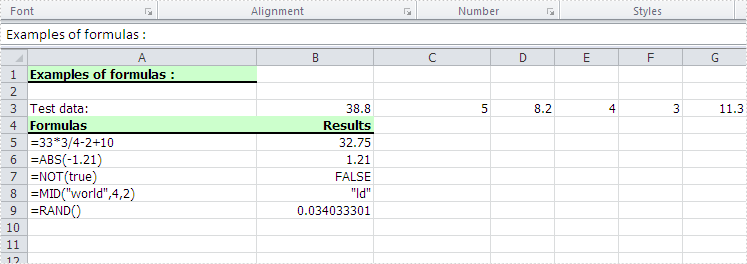Thursday, 28 July 2011 05:39

### Read Excel Formulas in C#, VB.NET

Written by support iceblue

#### Introduction

After we wrote formulas for Excel, the values would be displayed in specified cells. It is possible that the formulas are wrongly used or lost because there are too many formulas. Therefore, sometimes, we need to read the formula for one cell to ensure if the formula is right. Well then, how to read Excel formula?

### Read Formula in Microsoft Excel

Through Formulas tab in Excel 2007, we can find one button named Show Formulas. Select the cells which we want to read formulas, and then click the button. After that, we can see the formulas instead of values.

Besides using the button, we can also use keyboard shortcut to read formulas in Excel. Select the cells where we want to show the formulas, and then press Ctrl + ` to read.

### Read Excel Formulas via Spire.XLS

Spire.XLS presents you an easy way to read formula in the worksheet. You can get the formula through the value you provide, while you should specify where the value is. In the demo, we load a workbook from file named "ReadFormulaSample.xls" which has a formula written in the sheet["B5"], we can read the formula through its value in sheet["C5"]. In this example, in order to view, we read the formula to the sheet["D5"].

The following code displays the method to read formulas for cells with C#/VB.NET:

[C#]
```using Spire.Xls;

{
class Program
{
static void Main(string[] args)
{
//Create a new workbook
Workbook workbook = new Workbook();

//Initialize the worksheet
Worksheet sheet = workbook.Worksheets;

sheet.Range["D5"].Text = sheet.Range["C5"].Formula;

//Save the file
workbook.SaveToFile("sample.xls",ExcelVersion.Version97to2003);

//Launch the file
System.Diagnostics.Process.Start("Sample.xls");
}
}
```
[VB.NET]
```Imports Spire.Xls

Module Module1

Sub Main()
'Create a new workbook
Dim workbook As New Workbook()

'Initialize the worksheet
Dim sheet As Worksheet = workbook.Worksheets(0)

sheet.Range("D5").Text = sheet.Range("C5").Formula

'Save doc file.
workbook.SaveToFile("Sample.xls",ExcelVersion.Version97to2003)

'Launching the MS Word file.
System.Diagnostics.Process.Start("Sample.xls")
End Sub
End Module
```

After running the demo, you may find a formula appear in the worksheet you specify: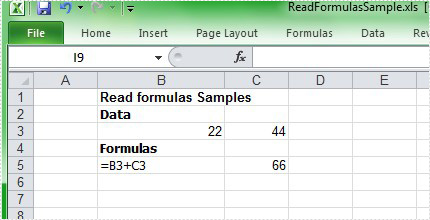Monday, 24 January 2011 09:43

### Add Excel Formulas in C#, VB.NET

In an Excel Worksheet, we may import a great deal of data. Sometimes, we need to calculate the data to get other numbers we need. It is a time consuming job when we deal with large spreadsheets and more complex data. Formula is the main tool to calculate data. There are various formulas included in Excel. Below I will show you how to add formula in C#, VB.NET by Spire.XLS.

Spire.XLS .NET is a Professional and stable .NET Excel component which enables developers/programmers to operate Excel files with their ASP.NET web sites and Windows Forms applications. It supports calculate complex Excel Formulas. Spire.XLS presents you an easiest way to add formula for Excel.

Please check the effective screenshot first.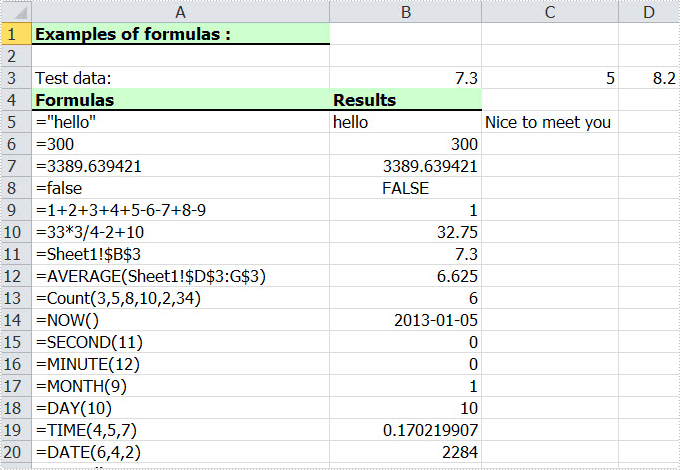Now we will give you a demo with many kinds of formulas written in the worksheet. The formula can be string, bool value, calculation, sheet area reference, time and so on. Here, we first reference a variable "currentRow" to control rows of all kinds of formulas. Second, reference "currentFormula". Assign value for Worksheet.Range [cellRange]. Text to write a formula, and then via currentFormula to assign value for Worksheet.Range [cellRange]. Formula to get the formula results.

[C#]
```using Spire.Xls;

namespace Formula
{
class Program
{
static void Main(string[] args)
{
//Create a new workbook
Workbook workbook = new Workbook();

//Initialize worksheet
Worksheet sheet = workbook.Worksheets;

//initialize currentRow
int currentRow = 3;
string currentFormula = string.Empty;

//test data
sheet.Range[currentRow, 2].NumberValue = 7.3;
sheet.Range[currentRow, 3].NumberValue = 5; ;
sheet.Range[currentRow, 4].NumberValue = 8.2;
sheet.Range[currentRow, 5].NumberValue = 4;
sheet.Range[currentRow, 6].NumberValue = 3;
sheet.Range[currentRow, 7].NumberValue = 11.3;

//string.
currentFormula = "=\"hello\"";
sheet.Range[++currentRow, 1].Text = "=\"hello\"";
sheet.Range[currentRow, 2].Formula = currentFormula;

//bool.
currentFormula = "=false";
sheet.Range[++currentRow, 1].Text = currentFormula;
sheet.Range[currentRow, 2].Formula = currentFormula;

//calculation
currentFormula = "=33*3/4-2+10";
sheet.Range[++currentRow, 1].Text = currentFormula;
sheet.Range[currentRow, 2].Formula = currentFormula;

//sheet area reference
currentFormula = "=AVERAGE(Sheet1!\$D\$3:G\$3)";
sheet.Range[++currentRow, 1].Text = currentFormula;
sheet.Range[currentRow, 2].Formula = currentFormula;

//time
currentFormula = "=NOW()";
sheet.Range[++currentRow, 1].Text = currentFormula;
sheet.Range[currentRow, 2].Formula = currentFormula;
sheet.Range[currentRow, 2].Style.NumberFormat = "yyyy-MM-DD";

//Save the file
workbook.SaveToFile("Sample.xls",ExcelVersion.Version97to2003);

//Launch the file
System.Diagnostics.Process.Start("Sample.xls");
}
}
}
```
[VB.NET]
```Imports Spire.Xls

Module Module1

Sub Main()
'Create a new workbook
Dim workbook As New Workbook()

'Initialize worksheet
Dim sheet As Worksheet = workbook.Worksheets(0)

'initialize currentRow
Dim currentRow As Integer = 3
Dim currentFormula As String = String.Empty

'test data
sheet.Range(currentRow, 2).NumberValue = 7.3
sheet.Range(currentRow, 3).NumberValue = 5

sheet.Range(currentRow, 4).NumberValue = 8.2
sheet.Range(currentRow, 5).NumberValue = 4
sheet.Range(currentRow, 6).NumberValue = 3
sheet.Range(currentRow, 7).NumberValue = 11.3

'string.
currentFormula = "=""hello"""
sheet.Range(currentRow, 2).Formula = currentFormula

'bool.
currentFormula = "=false"
sheet.Range(currentRow, 2).Formula = currentFormula

'calculation
currentFormula = "=33*3/4-2+10"
sheet.Range(currentRow, 2).Formula = currentFormula

'sheet area reference
currentFormula = "=AVERAGE(Sheet1!\$D\$3:G\$3)"
sheet.Range(currentRow, 2).Formula = currentFormula

'time
currentFormula = "=NOW()"
sheet.Range(currentRow, 2).Formula = currentFormula
sheet.Range(currentRow, 2).Style.NumberFormat = "yyyy-MM-DD"

'Save doc file.
workbook.SaveToFile("Sample.xls",ExcelVersion.Version97to2003)

'Launching the MS Word file.
System.Diagnostics.Process.Start("Sample.xls")

End Sub
End Module
```

Spire.XLS supports many methods to add a formula for Excel including different parts. As a professional .NET Excel component, it owns the ability of inserting content into Excel document, formatting cells and converting Excel documents to popular office file formats. Spire.XLS for WPF supports Excel 97-2003, Excel 2007 and Excel 2010.